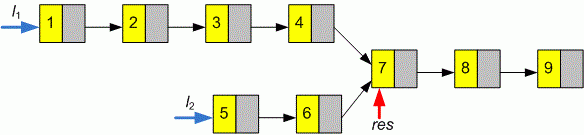bolt
Try our new interface for solving problems
Problems

Time limit 1 second
Memory limit 128 MiB

Find the point of intersection of two singly linked lists. Return the pointer to the node where the intersection of two lists starts.

Definition of a single linked list:

// Java
class ListNode {
int val;
ListNode next;
ListNode(int x) {
val = x;
next = null;
}
}

// C++
class ListNode
{
public:
int val;
ListNode *next;
ListNode(int x) : val(x), next(NULL) {}
};

// C
struct ListNode
{
int val;
struct ListNode *next;
};


Implement a function intersection that returns a pointer to the node where the intersection of two singly linked lists starts.

// Java
ListNode intersection(ListNode l1, ListNode l2)

// C, C++
ListNode* intersection(ListNode *l1, ListNode *l2)


#### ExampleFunction intersection must return a pointer to the node with value 7.

Author Mykhailo Medvediev